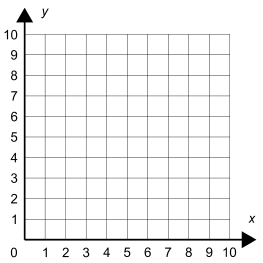# Parallel and Perpendicular Lines

Directions: Using the digits 0 to 9, at most once each time, fill in blanks to create a set of 4 points that create either parallel or perpendicular lines, depending on how you connect them.( ___, ___ ) ( ___, ___ ) ( ___, ___ ) ( ___, ___ )

### Hint

What makes lines either parallel or perpendicular?

What is that relationship?

There are various possible answers, one is:
(3,0) (2,4) (6,5) (7,1)

Source: Bryan Anderson

## Exponential Powers

Directions: Using the digits 0 to 9 at most one time each, place a digit …

1.(5,5) (5,10) (7,5) (7,10)

•(5,5) (6,10) (4,5) (7,10)

2.(6, 14) (6,10) (8, 13) (8,11)

3.(1,3) (8,9) (0,7) (5,2) Perpendicular.

4.(5,5) (6,6) (4,7) (7,4)